# Machine Learning Algorithms

India’s #1 Machine Learning Course

Machine Learning is a sub field of computer science and Information Technology that focuses on data analysis using various algorithms and tools. In this article, we will cover machine learning algorithms and their practical use cases. If you are a student of data science or data analytics, you may be more interested to learn a deep understanding of Machine Learning concepts so let us take a deep dive into our topic.

Nowadays there is a huge demand of machine learning and artificial Intelligence professionals who can work in top-level IT organizations and as per the market research and survey,only 10% candidates are eligible in this domain so you can start your career in this field but you should have practical understanding of all machine learning concepts and its algorithms.

“Machine Learning” ? What it will do ? so we use  machine learning algorithms to make predictions for example “what would be the growth of sales rate in 2021 and 2022 or “Are you eligible to take loan or not”.professionals use various algorithms to complete such task that we will cover in this article.

The Basic Fundamental concept of machine learning is to divide data sets into two part first training datasets and second test datasets so professionals use training datasets to create machine learning models and  finally ,use test  datasets to make predictions.

##### Linear Regression in Machine Learning.

The goal of Linear Regression is the find the best fit line in the given datasets and using this line be can predict future value so you need to calculate slope of this line and intercept of the line.now the question is how you can say that your line fits best in the the given datasets so we calculate SSE that is sum of square error and this SSE should be minimum then you can say your line fits best for a given data set.

Let us say we have two variable  x (area in sq ft)  and y (price of a flat)
x= { 1,2,3}
y= {3,2,4}

SSE = (( mx+b) – y’) power 2
where mx+b is predicted value and y’ is actual value

SSE = ( m*1+b-3) power 2+(m*2+b-2) power 2+ (m*3+b-4) power 2
After taking derivative now.
de/dm= 2* ( m*1+b-3) +2* (m*2+b-2)* 2+  2* (m*3+b-4) *3

=28m+12b-38=0

de/db= 2* ( m*1+b-3) +2* (m*2+b-2)+  2* (m*3+b-4)

=12m+6b-18=0

m=1/2 and b=2

Example:

Linear Line Eq: y=mx+b

so now our equation is y=x/2+2 that will predict y values.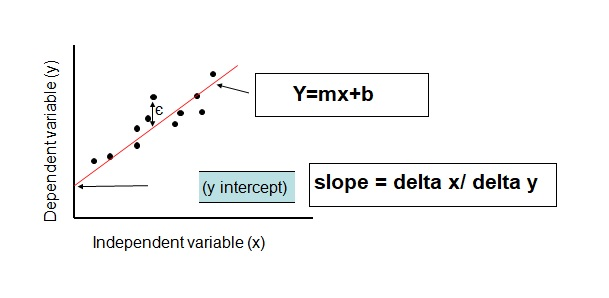##### Decision Tree Machine Learning algorithm

As the name suggested it is a tree based model that is used to take decisions and most common tool in machine learning algorithms and it can be used in the classification and regression both and if it is used for classification then we will say it is a tree structured classifier so let us take an example to understand the process of a decision tree model. say a bank want to predict that a candidate is eligible for a loan or not based on their credit score.

Training Data Set—->ML ALGORITHM—–>Generate a Classifier ( Decision Tree)  <—–New Tuple

So,when we will produce a new input tuple into our classifier then our classifier tells us about the class in which that tuple( row) belongs. In the decision tree we have two types of nodes first decision node and second a leaf node.using decision nodes you classify that which thing belong to which category and gets classification at the leaf node.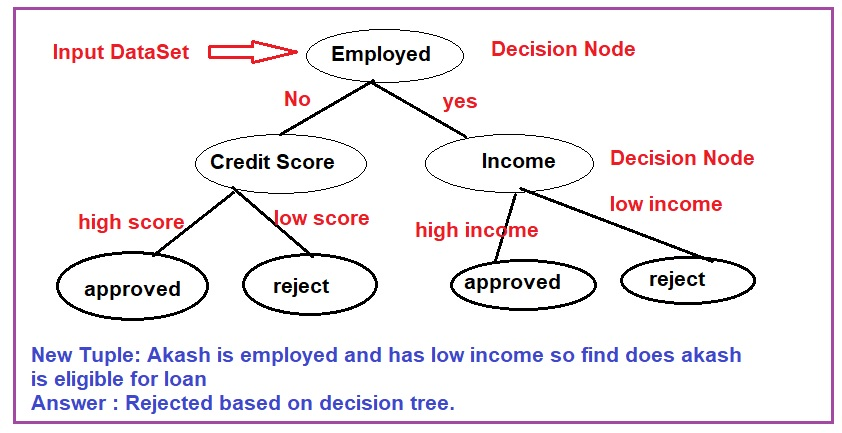In the above diagram our decision tree model is ready so we can pass any new data set into our model to classify that which employee belongs to which category or which candidate is eligible for loan.

##### Logistic Regression in Machine Learning.

Logistic Regression is a part of classification that gives answer in binary format like yes or no , true and false and 0 or 1.In this machine learning models we have two types of variables first is dependent variable and second is independent variable,say dependent variable is y that we want to predict and independent variable is x that is given so in this techniques dependent variable outcome is always binary and x can be continues or binary. this model is used to find the probability between x and y.

Logistic Regression produced a probability value and using this value we predict outcome in binary form. probability is possibility for a task to be done like “Does it rain today or not and what would be the possibility to rain today.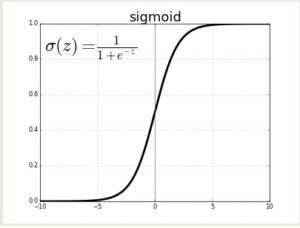Logistic Regression is used to find an email is spam or not and it use learning rate parameter that is a weight between 1 and 0 and changing during the logistic regression process so it change our model time to time to get minimum error and best predictions.

##### Naive Bayes Classifier Algorithm.

Naive Bayes algorithms work as a classifier that is use to classify a class based on some probability value.the goal of naive Bayes is to find the posterior probability p ( A|B) using formula  P (A|B) = [p (B |A) * P (A) ] /  p ( B) where p stands for probability of a given variable.Naive Bayes is widely used algorithm in machine learning and the fundamental idea of this algorithm is to find frequency table for each attribute with respect to the target.

Let us take an Example say we want to calculate the name of a fruit that is “yellow”, “sweet” and “long” from a given table listed below.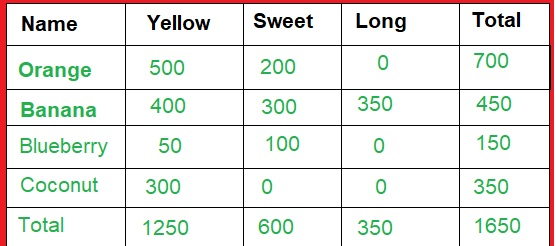p ( A|B) means find the probability of A when Probability of B is given and it is true.

First Find :
P ( yellow | orange )= [ p ( orange |yellow ) * P(yellow) ]/ p(orange)

P ( yellow | orange ) =[500/1250 * 1250/1650]/ (700/1200)

Second Find:

P ( sweet |orange) = [p (orange | sweet)* p(sweet)] / p( orange)

Third find :

p ( long | orange) = [p(orange | long)* p (long) ] / p (orange)

Last Find:

p ( Name | Orange ) = P ( yellow | orange ) * P ( sweet |orange) * p ( long | orange)

So Now you need to follow the above steps for “banana” ,”blueberry” and “coconut” and find the highest probability so the maximum probability value would be your answer.

##### Finding Euclidean Distance between two points P and Q.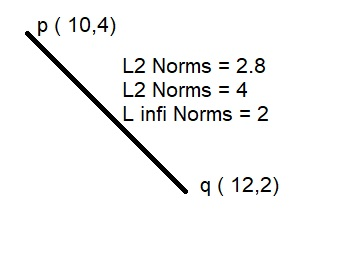Say p = ( 10,4) and Q=(12,2) where x1=10,y1=4 and x2=12 ,y2=2

L2 norms = root of [ square of (x1-x2) + square of (y1-y2)]
L1 norms = | x1-x2 | + | y1-y2|
L (infinitive) norms = max (|x1-x2|,|y1-y2|)

L2-norms = root of [square of (10-12)+square of (4-2)] =2.8
L1 norms = |10-12| + |4-2| = 4
L (infinitive) norms = max ( |-2|,|2|) = 2#### Jashika Bhatt

Jashika is a professional blogger, self-published author and speaker at techbook and passionate about digital marketing.